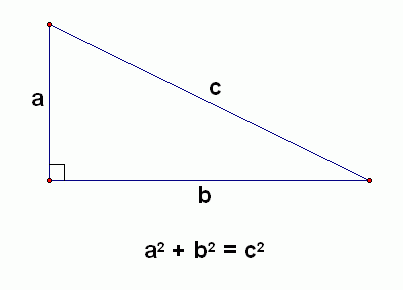# Babylonian Math

Until I can locate my camera’s power cord, there will be no vacation photos. Try to restrain your disappointment. ;)

While the kids were at Vacation Bible School yesterday (a concept I find simultaneously delightful and mystifying given my secular upbringing — but that’s a whole other post) I spent an hour avoiding cleaning the house and instead watching the first two lectures in a series devoted to the history of mathematics.

I’ve really come around to liking math lately. It’s a foreign language I never bothered to learn well when I was in school. I’m slowly fixing that.

I especially like learning about ancient math and science. It’s riveting to discover that people 4,000 years ago were far more advanced and modern in thinking than I’d realized. The ancient Babylonians predicted eclipses and planetary alignments centuries into the future, they calculated the square root of 2 to six decimal places, they figured out a geometrical solution to quadratic equations, and they used the Pythagorean theorem 1,500 years before Pythagoras was born.

I wish I had learned in school that algebra is shorthand for something that can be solved using geometry — visually. And had been shown those visuals. I think it would have been a lot easier to grasp.

Take the Pythagorean theorem. The algebraic equation iswhere a and b represent two sides of a right triangle and c is the hypotenuse. When we visualize it, this is probably what we see:It doesn’t really tell us much.

But what if we think about it like this: a square with sides of length A will have an area that, when added to the area of a square with the sides of length B, will equal the area of a square with the sides of length C.

We’ll use the classic 3/4/5 triangle:This makes more sense to me. And it’s how the Babylonians derived the theorem themselves. When the numbers of the three sides are all whole numbers we call them Pythagorean triples. The Babylonians calculated this triple: 4601 (squared) + 4800 (squared) = 6649 (squared). Crazy.

How about quadratic equations? Let’s say we’re staring at this quadratic equation in 10th-grade algebra and trying to figure out how to solve it:

x² + 2x = 15

Before this concept could be represented algebraically, the Babylonians figured it out geometrically. Let’s say you wanted to construct a rectangular building and you knew you only had 15 square units of ground space to work with. You know that you’d like to divide it into two rooms, and one of those rooms needs to have a wall that is 2 units long. The other room needs to be a square. How long will the walls of the square room be? You can draw it like this:Can you see it?  x² + 2x = 15

Now you can split the rectangle with a known side of 2 units into equal pieces:Next you move these two pieces around like this:Now comes the part known as “completing the square”. You create a little square to fill in the missing space in the bigger square. We can tell from the drawing that the new square will be 1 unit by 1 unit.

You’re going to need to add an equal square to the other side to keep the equation equal:The little square has an area of 1 unit. We can think of the big square this way: its area is the same as 15 plus 1. We can write it out showing that one side multiplied by the other side equals 15 plus 1:

(x + 1)(x + 1) = 15 + 1

We’ll write it out a new way:

(x + 1)² = 16

And now we need to take the square root of each side, something the Babylonians were good at doing.

(Square root, by the way, is the length of a side of the square that can be divided into equal squares of a given number. So 16 little equal squares can be combined to form a larger square with sides the length of 4. The square root of 16 is therefore 4. This becomes more complicated to do geometrically when you’re not using whole numbers, like say, finding the square root of 15. But it works for square roots that are whole numbers.)

Anyway, taking the square root of each side gives us:

x + 1 = 4

Now it’s simple to solve for the unknown. Simply subtract 1 from each side and:

x = 3

Now we know our square room is 3 by 3 units, and the total rectangle building is 3 by 5 units.

Algebraically speaking, x also could equal -5, but I don’t know if the Babylonians could calculate that. While I’m aware that there must be some use for -5 as an answer to this equation, it isn’t practical for the question I posed above about building dimensions, so it doesn’t much bother me whether the Babylonians could calculate it or not.

When I learned how to solve equations like this in high school, we did what is called “factoring”, meaning that you have to figure out what numbers can be multiplied together to get 15, and then insert them, one at a time, into the equation. One would be positive and the other negative, and so you would reason out 3 and -5 after trying them in the equation. It seemed so arbitrary to me at the time, and I had completely forgotten how to do it at all until I looked it up on the internet.

But I think I can remember how to complete the square. Pretty neat, huh?

Categories: Brain Workouts